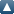# Items where Author is "Mohanty, RK"Up a level
 Export as ASCII CitationBibTeXDublin CoreEP3 XMLEndNoteHTML CitationJSONMETSMultiline CSVObject IDsOpenURL ContextObjectRDF+N-TriplesRDF+N3RDF+XMLRefWorksReferReference Manager
Group by: Item Type | No Grouping
Number of items: 16.

## Journal article

MOHANTY, R.K. and EVANS, D.J., 2005. Alternating group explicit parallel algorithms for the solution of one-space dimensional non-linear singular parabolic equations using an O(k(2)+h(4)) difference method. International Journal of Computer Mathematics, 82 (2), pp. 203-218. ISSN 0020-7160

MOHANTY, R.K., EVANS, D.J. and ARORA, U., 2005. Convergent spline in tension methods for singularly perturbed two-point singular boundary value problems. International Journal of Computer Mathematics, 82 (1), pp. 55-66. ISSN 0020-7160

MOHANTY, R.K. and EVANS, D.J., 2005. Highly accurate two parameter CAGE parallel algorithms for non-linear singular two point boundary value problems. International Journal of Computer Mathematics, 82 (4), pp. 433-444. ISSN 0020-7160

EVANS, D.J. and MOHANTY, R.K., 2005. On the application of the SMAGE parallel algorithms on a non-uniform mesh for the solution of non-linear two-point boundary value problems with singularity. International Journal of Computer Mathematics, 82 (3), pp. 341-353. ISSN 0020-7160

MOHANTY, R.K. and EVANS, D.J., 2004. Fourth-order accurate BLAGE iterative method for the solution of two-dimensional elliptic equations in polar co-ordinates. International Journal of Computer Mathematics, 81 (12), pp. 1537-1548. ISSN 0020-7160

MOHANTY, R.K., JHA, N. and EVANS, D.J., 2004. Spline in compression method for the numerical solution of singularly perturbed two-point singular boundary-value problems. International Journal of Computer Mathematics, 81 (5), pp. 615-627. ISSN 0020-7160

MOHANTY, R.K., EVANS, D.J. and KUMAR, D., 2003. High accuracy difference formulae for a fourth order quasi-linear parabolic initial boundary value problem of first kind. International Journal of Computer Mathematics, 80 (3), pp. 381-398. ISSN 0020-7160

MOHANTY, R.K. and EVANS, D.J., 2003. A fourth order accurate cubic spline alternating group explicit method for non-linear singular two point boundary value problems. International Journal of Computer Mathematics, 80 (4), pp. 479-492. ISSN 0020-7160

MOHANTY, R.K. and EVANS, D.J., 2003. The numerical solution of fourth order mildly quasi-linear parabolic initial boundary value problem of second kind. International Journal of Computer Mathematics, 80 (9), pp. 1147-1159. ISSN 0020-7160

EVANS, D.J. and MOHANTY, R.K., 2002. Alternating group explicit method for the numerical solution of non-linear singular two-point boundary value problems using a fourth order finite difference method. International Journal of Computer Mathematics, 79 (10), pp. 1121-1133. ISSN 0020-7160

MOHANTY, R.K., EVANS, D.J. and PANDEY, P.K., 2001. Block iterative methods for the numerical solution of three dimensional mildly non-linear biharmonic problems of first kind. International Journal of Computer Mathematics, 77 (2), pp. 319-332. ISSN 0020-7160

MOHANTY, R.K., ARORA, U. and JAIN, M.K., 2001. Fourth-order approximation for the three space dimensional certain mildly quasi-linear hyperbolic equation. Numerical Methods for Partial Differential Equations, 17 (3), pp. 277-289. ISSN 0749-159X

MOHANTY, R.K., ARORA, U. and JAIN, M.K., 2001. Linear stability analysis and fourth-order approximations at first time level for the two space dimensional mildly quasi-linear hyperbolic equations. Numerical Methods for Partial Differential Equations, 17 (6), pp. 607-618. ISSN 0749-159X

MOHANTY, R.K., JAIN, M.K. and KUMAR, D., 2001. Single cell discretization of O(kh(2)+h(4)) for the estimates of partial derivative u/partial derivative n for two-space dimensional quasi-linear parabolic equation. Numerical Methods for Partial Differential Equations, 17 (3), pp. 250-261. ISSN 0749-159X

MOHANTY, R.K., EVANS, D.J. and DEY, S., 2001. Three point discretization of order four and six for (du/dx) of the solution of non-linear singular two point boundary value problem. International Journal of Computer Mathematics, 78 (1), pp. 123-139. ISSN 0020-7160

MOHANTY, R.K. and JAIN, M.K., 2001. An unconditionally stable alternating direction implicit scheme for the two space dimensional linear hyperbolic equation. Numerical Methods for Partial Differential Equations, 17 (6), pp. 684-688. ISSN 0749-159X

This list was generated on Tue Oct 19 11:18:26 2021 UTC.